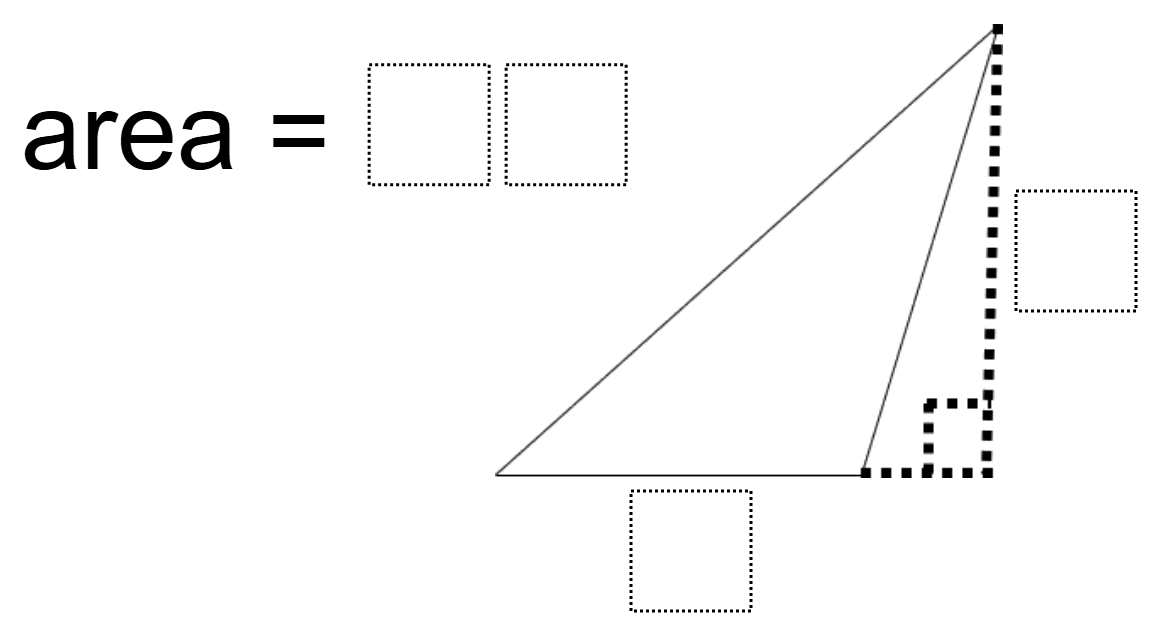# Area of an Obtuse Triangle

Directions: Using the digits 1 to 9 at most one time each, place a digit in each box to make a triangle with side lengths that give the corresponding area.### Hint

How do you calculate the area of a triangle?

Multiple Answers. A base of 3, a height of 8, and an area of 12. A base of 4, a height 9, and an area of 18. A base of 6, a height of 9 and an area of 27. A base of 8, a height of 9, and an area of 36.

Source: Owen Kaplinsky

## Greatest Common Factor

Directions: Using the digits 0 to 9 at most one time each, place a digit …

1.2.